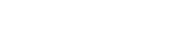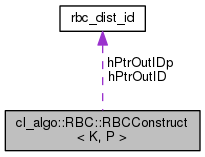RandomBallCover  1.2.1 Hosted by GitHubcl_algo::RBC::RBCConstruct< K, P > Class Template Reference

Interface class for constructing the Random Ball Cover data structure. More...

#include <algorithms.hpp>

Collaboration diagram for cl_algo::RBC::RBCConstruct< K, P >:[legend]

## Public Types

enum  Memory : uint8_t {
Memory::H_IN_X, Memory::H_IN_R, Memory::H_OUT_ID, Memory::H_OUT_RNK,
Memory::H_OUT_N, Memory::H_OUT_O, Memory::H_OUT_X_P, Memory::H_OUT_ID_P,
Memory::D_IN_X, Memory::D_IN_R, Memory::D_OUT_D, Memory::D_OUT_ID,
Memory::D_OUT_RNK, Memory::D_OUT_N, Memory::D_OUT_O, Memory::D_OUT_X_P,
Memory::D_OUT_ID_P
}
Enumerates the memory objects handled by the class. More...

## Public Member Functions

RBCConstruct (clutils::CLEnv &_env, clutils::CLEnvInfo< 1 > _info)
Configures an OpenCL environment as specified by _info. More...

cl::Memory & get (RBCConstruct::Memory mem)
Returns a reference to an internal memory object. More...

void init (unsigned int _nx, unsigned int _nr, unsigned int _d, float _a=1.f, int _permID=0, Staging _staging=Staging::IO)
Configures kernel execution parameters. More...

void write (RBCConstruct::Memory mem=RBCConstruct::Memory::D_IN_X, void *ptr=nullptr, bool block=CL_FALSE, const std::vector< cl::Event > *events=nullptr, cl::Event *event=nullptr)
Performs a data transfer to a device buffer. More...

void * read (RBCConstruct::Memory mem=RBCConstruct::Memory::H_OUT_X_P, bool block=CL_TRUE, const std::vector< cl::Event > *events=nullptr, cl::Event *event=nullptr)
Performs a data transfer to a staging buffer. More...

void run (const std::vector< cl::Event > *events=nullptr, cl::Event *event=nullptr)
Executes the necessary kernels. More...

float getAlpha ()
Gets the scaling factor $$\alpha$$. More...

void setAlpha (float _a)
Sets the scaling factor $$\alpha$$. More...

template<typename period >
double run (clutils::GPUTimer< period > &timer, const std::vector< cl::Event > *events=nullptr)
Executes the necessary kernels. More...

## Public Attributes

cl_float * hPtrInX

cl_float * hPtrInR

rbc_dist_idhPtrOutID

cl_uint * hPtrOutRnk

cl_uint * hPtrOutN

cl_uint * hPtrOutO

cl_float * hPtrOutXp

rbc_dist_idhPtrOutIDp

## Detailed Description

### template<KernelTypeC K, RBCPermuteConfig P> class cl_algo::RBC::RBCConstruct< K, P >

Interface class for constructing the Random Ball Cover data structure.

The process involves finding the representative lists and permuting the database points to form the lists within the database. The RBC data structure built is the one described in the exact search algorithm. For more details, see here.

Note
The kernels used in this process are available in kernels/rbc_kernels.cl and kernels/scan_kernels.cl.
The class creates its own buffers. If you would like to provide your own buffers, call get to get references to the placeholders within the class and assign them to your buffers. You will have to do this strictly before the call to init. You can also call get (after the call to init) to get a reference to a buffer within the class and assign it to another kernel class instance further down in your task pipeline.

The following input/output OpenCL memory objects are created by a RBCConstruct instance:

Name Type Placement I/O Use Properties Size
H_IN_X Buffer Host I Staging CL_MEM_READ_WRITE $$n_x*d *sizeof\ (cl\_float)$$
H_IN_R Buffer Host I Staging CL_MEM_READ_WRITE $$n_r*d *sizeof\ (cl\_float)$$
H_OUT_ID Buffer Host O Staging CL_MEM_READ_WRITE $$n_x*sizeof\ (rbc\_dist\_id)$$
H_OUT_RNK Buffer Host O Staging CL_MEM_READ_WRITE $$n_x *sizeof\ (cl\_uint)$$
H_OUT_N Buffer Host O Staging CL_MEM_READ_WRITE $$n_r *sizeof\ (cl\_uint)$$
H_OUT_O Buffer Host O Staging CL_MEM_READ_WRITE $$n_r *sizeof\ (cl\_uint)$$
H_OUT_X_P Buffer Host O Staging CL_MEM_READ_WRITE $$n_x*d *sizeof\ (cl\_float)$$
H_OUT_ID_PBuffer Host O Staging CL_MEM_READ_WRITE $$n_x*sizeof\ (rbc\_dist\_id)$$
D_IN_X Buffer Device I Processing CL_MEM_READ_ONLY $$n_x*d *sizeof\ (cl\_float)$$
D_IN_R Buffer Device I Processing CL_MEM_READ_ONLY $$n_r*d *sizeof\ (cl\_float)$$
D_OUT_ID Buffer Device O Processing CL_MEM_READ_WRITE $$n_x*sizeof\ (rbc\_dist\_id)$$
D_OUT_RNK Buffer Device O Processing CL_MEM_READ_WRITE $$n_x *sizeof\ (cl\_uint)$$
D_OUT_N Buffer Device O Processing CL_MEM_READ_WRITE $$n_r *sizeof\ (cl\_uint)$$
D_OUT_O Buffer Device O Processing CL_MEM_READ_WRITE $$n_r *sizeof\ (cl\_uint)$$
D_OUT_X_P Buffer Device O Processing CL_MEM_READ_WRITE $$n_x*d *sizeof\ (cl\_float)$$
D_OUT_ID_PBuffer Device O Processing CL_MEM_READ_WRITE $$n_x*sizeof\ (rbc\_dist\_id)$$
Template Parameters
 K configures the class for using one of the available kernels for computing the point distances. P configures the class for using one of the available kernels for the database permutation.

## Member Enumeration Documentation

template<KernelTypeC K, RBCPermuteConfig P>
 enum cl_algo::RBC::RBCConstruct::Memory : uint8_t
strong

Enumerates the memory objects handled by the class.

Note
H_* names refer to staging buffers on the host.
D_* names refer to buffers on the device.
Enumerator
H_IN_X

Input staging buffer for the database.

H_IN_R

Input staging buffer for the representatives.

H_OUT_ID

Output staging buffer with the representative ids for each db point.

H_OUT_RNK

Output staging buffer with the indices of the points within each rep. list.

H_OUT_N

Output staging buffer with the cardinalities of the representative lists.

H_OUT_O

Output staging buffer with the offsets of the representative lists within the db.

H_OUT_X_P

Output staging buffer for the permuted database.

H_OUT_ID_P

Output staging buffer with the representative ids for each point in the permuted db.

D_IN_X

Input buffer for the database.

D_IN_R

Input buffer for the representatives.

D_OUT_D

Output buffer for the array of distances.

D_OUT_ID

Output buffer with the representative ids for each db point.

D_OUT_RNK

Output buffer with the indices of the points within each rep. list.

D_OUT_N

Output buffer with the cardinalities of the representative lists.

D_OUT_O

Output buffer with the offsets of the representative lists within the db.

D_OUT_X_P

Output buffer for the permuted database.

D_OUT_ID_P

Output buffer with the representative ids for each point in the permuted db.

## Constructor & Destructor Documentation

template<KernelTypeC K, RBCPermuteConfig P>
 cl_algo::RBC::RBCConstruct< K, P >::RBCConstruct ( clutils::CLEnv & _env, clutils::CLEnvInfo< 1 > _info )

Configures an OpenCL environment as specified by _info.

Parameters
 [in] _env opencl environment. [in] _info opencl configuration. Specifies the context, queue, etc, to be used.

## Member Function Documentation

template<KernelTypeC K, RBCPermuteConfig P>
 cl::Memory & cl_algo::RBC::RBCConstruct< K, P >::get ( RBCConstruct< K, P >::Memory mem )

Returns a reference to an internal memory object.

This interface exists to allow CL memory sharing between different kernels.

Parameters
 [in] mem enumeration value specifying the requested memory object.
Returns
A reference to the requested memory object.
template<KernelTypeC K, RBCPermuteConfig P>
 float cl_algo::RBC::RBCConstruct< K, P >::getAlpha ( )

Gets the scaling factor $$\alpha$$.

Note
If the template parameter, K, has the value SHARED_NONE, SHARED_R, or SHARED_X_R, the parameter $$\alpha$$ is not applicable and the value returned is $$\infty$$.
Returns
The scaling factor $$\alpha$$.
template<KernelTypeC K, RBCPermuteConfig P>
 void cl_algo::RBC::RBCConstruct< K, P >::init ( unsigned int _nx, unsigned int _nr, unsigned int _d, float _a = 1.f, int _permID = 0, Staging _staging = Staging::IO )

Configures kernel execution parameters.

Sets up memory objects as necessary, and defines the kernel workspaces.

Note
If you have assigned a memory object to one member variable of the class before the call to init, then that memory will be maintained. Otherwise, a new memory object will be created.
Parameters
 [in] _nx number of database points. [in] _nr number of representative points. [in] _d dimensionality of the associated points. [in] _a factor scaling the results of the distance calculations for the geometric $$x_g$$ and photometric $$x_p$$ dimensions of the $$x\epsilon\mathbb{R}^8$$ points. That is, $$\|x-x'\|_2^2= f_g(a)\|x_g-x'_g\|_2^2+f_p(a)\|x_p-x'_p\|_2^2$$. This parameter is applicable when involving the "Kinect" kernels. That is, when the template parameter, K, gets the value KINECT, KINECT_R, or KINECT_X_R. For more details, take a look at euclideanSquaredMetric8 in kernels/rbc_kernels.cl. [in] _permID flag to indicate whether or not to also permute the ID array. [in] _staging flag to indicate whether or not to instantiate the staging buffers.
template<KernelTypeC K, RBCPermuteConfig P>
 void * cl_algo::RBC::RBCConstruct< K, P >::read ( RBCConstruct< K, P >::Memory mem = RBCConstruct< K, P >::Memory::H_OUT_X_P, bool block = CL_TRUE, const std::vector< cl::Event > * events = nullptr, cl::Event * event = nullptr )

Performs a data transfer to a staging buffer.

The transfer happens from a device buffer to the associated (specified) staging buffer on the host.

Parameters
 [in] mem enumeration value specifying an output staging buffer. [in] block a flag to indicate whether to perform a blocking or a non-blocking operation. [in] events a wait-list of events. [out] event event associated with the read operation to the staging buffer.
template<KernelTypeC K, RBCPermuteConfig P>
 void cl_algo::RBC::RBCConstruct< K, P >::run ( const std::vector< cl::Event > * events = nullptr, cl::Event * event = nullptr )

Executes the necessary kernels.

The function call is non-blocking.

Parameters
 [in] events a wait-list of events. [out] event event associated with the last kernel execution.
template<KernelTypeC K, RBCPermuteConfig P>
template<typename period >
 double cl_algo::RBC::RBCConstruct< K, P >::run ( clutils::GPUTimer< period > & timer, const std::vector< cl::Event > * events = nullptr )
inline

Executes the necessary kernels.

This run instance is used for profiling.

Parameters
 [in] timer GPUTimer that does the profiling of the kernel executions. [in] events a wait-list of events.
Returns
Τhe total execution time measured by the timer.
template<KernelTypeC K, RBCPermuteConfig P>
 void cl_algo::RBC::RBCConstruct< K, P >::setAlpha ( float _a )

Sets the scaling factor $$\alpha$$.

Updates the kernel argument for the scaling factor $$\alpha$$.

Note
If the template parameter, K, has the value SHARED_NONE, SHARED_R, or SHARED_X_R, the parameter $$\alpha$$ is not applicable and the function has no effect.
Parameters
 [in] _a scaling factor $$\alpha$$.
template<KernelTypeC K, RBCPermuteConfig P>
 void cl_algo::RBC::RBCConstruct< K, P >::write ( RBCConstruct< K, P >::Memory mem = RBCConstruct< K, P >::Memory::D_IN_X, void * ptr = nullptr, bool block = CL_FALSE, const std::vector< cl::Event > * events = nullptr, cl::Event * event = nullptr )

Performs a data transfer to a device buffer.

The transfer happens from a staging buffer on the host to the associated (specified) device buffer.

Parameters
 [in] mem enumeration value specifying an input device buffer. [in] ptr a pointer to an array holding input data. If not NULL, the data from ptr will be copied to the associated staging buffer. [in] block a flag to indicate whether to perform a blocking or a non-blocking operation. [in] events a wait-list of events. [out] event event associated with the write operation to the device buffer.

## Member Data Documentation

template<KernelTypeC K, RBCPermuteConfig P>
 cl_float* cl_algo::RBC::RBCConstruct< K, P >::hPtrInR

Mapping of the input staging buffer for the representatives.

template<KernelTypeC K, RBCPermuteConfig P>
 cl_float* cl_algo::RBC::RBCConstruct< K, P >::hPtrInX

Mapping of the input staging buffer for the database.

template<KernelTypeC K, RBCPermuteConfig P>
 rbc_dist_id* cl_algo::RBC::RBCConstruct< K, P >::hPtrOutID

Mapping of the output staging buffer with the representative ids for each point.

template<KernelTypeC K, RBCPermuteConfig P>
 rbc_dist_id* cl_algo::RBC::RBCConstruct< K, P >::hPtrOutIDp

Mapping of the output staging buffer with the representative ids for each point in the permuted db.

template<KernelTypeC K, RBCPermuteConfig P>
 cl_uint* cl_algo::RBC::RBCConstruct< K, P >::hPtrOutN

Mapping of the output staging buffer with the cardinalities of the representative lists.

template<KernelTypeC K, RBCPermuteConfig P>
 cl_uint* cl_algo::RBC::RBCConstruct< K, P >::hPtrOutO

Mapping of the output staging buffer with the offsets of the representative lists within the db.

template<KernelTypeC K, RBCPermuteConfig P>
 cl_uint* cl_algo::RBC::RBCConstruct< K, P >::hPtrOutRnk

Mapping of the output staging buffer with the indices of the points within each rep. list.

template<KernelTypeC K, RBCPermuteConfig P>
 cl_float* cl_algo::RBC::RBCConstruct< K, P >::hPtrOutXp

Mapping of the output staging buffer for the permuted database.

The documentation for this class was generated from the following files: